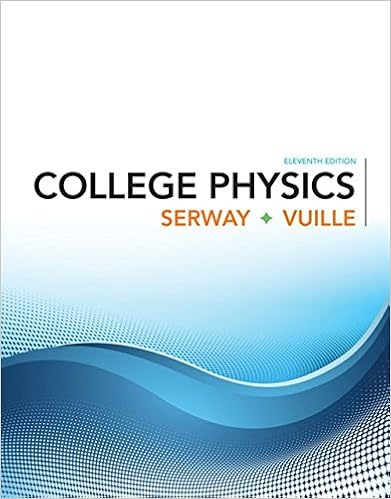# A ball is tied to the end of a rope of negligible

• Test Prep
• 9
• 100% (2) 2 out of 2 people found this document helpful

This preview shows page 2 - 5 out of 9 pages.

##### We have textbook solutions for you!
The document you are viewing contains questions related to this textbook.The document you are viewing contains questions related to this textbook.
Chapter 13 / Exercise 19
College Physics
Serway/VuilleExpert Verified
3. (6 points) A ball is tied to the end of a rope of negligible mass. The ball is spun at constant speed in acircle of radius 2.00 m, making 7.00 revolutions every 10.0 seconds. What is the magnitude of theacceleration of the ball?A) 67.9 m/s2B) 38.7 m/s2C) 29.3 m/s2D) 14.8 m/s2E) 74.2 m/s2
4. (6 points) In the figure, a ball is thrown up onto a roof, landingsome time later at heighth= 20 m above and distanced= 30 mhorizontally from the release point. The ball’s speed when itreaches its highest point is 10 m/s. How long does the ball take toland on the roof, from the time it leaves the ground?
This study resource wasshared via CourseHero.com
##### We have textbook solutions for you!
The document you are viewing contains questions related to this textbook.The document you are viewing contains questions related to this textbook.
Chapter 13 / Exercise 19
College Physics
Serway/VuilleExpert Verified
5. (6 points) Three blocks (A, B, C), each of mass M, areconnected by massless strings as shown. Block C is pulledto the right by a force that causes the entire system toaccelerate. Neglecting friction, thetensionin the stringconnecting block B and block C is:
6. (6 points) A student of mass 70.0 kg rides a Ferris wheel which rotates at aconstant speed. At thehighest point, the magnitude of the normal force on thestudent from the seat is!%= 565 N. What is the magnitude of the wheel’sacceleration?
7. (6 points) A forcePacts on a block of mass 2.0 kg. Initially,the block is at rest on the inclined plane (at+= 20o). Thecoefficient of static friction between the block and the plane is0.5, and the coefficient of kinetic friction is 0.3. If the magnitudeofPis 5N, what is the magnitude of acceleration of the block?A) 0 m/s2B) 1.6 m/s2C) 2.0 m/s2D) 2.9 m/s2E) 3.1 m/s
2
8. (6 points) As shown in the figure, a box of massm= 6.5 kgresting on a frictionless incline of angle, = 30.is connected bya cord that runs over a (frictionless and massless) pulley to amassless spring of spring constantk= 180 N/m. The spring is inits relaxed position when the box is released from rest. How fardown along the incline from its point of release does the boxslide before momentarily stopping?
•••